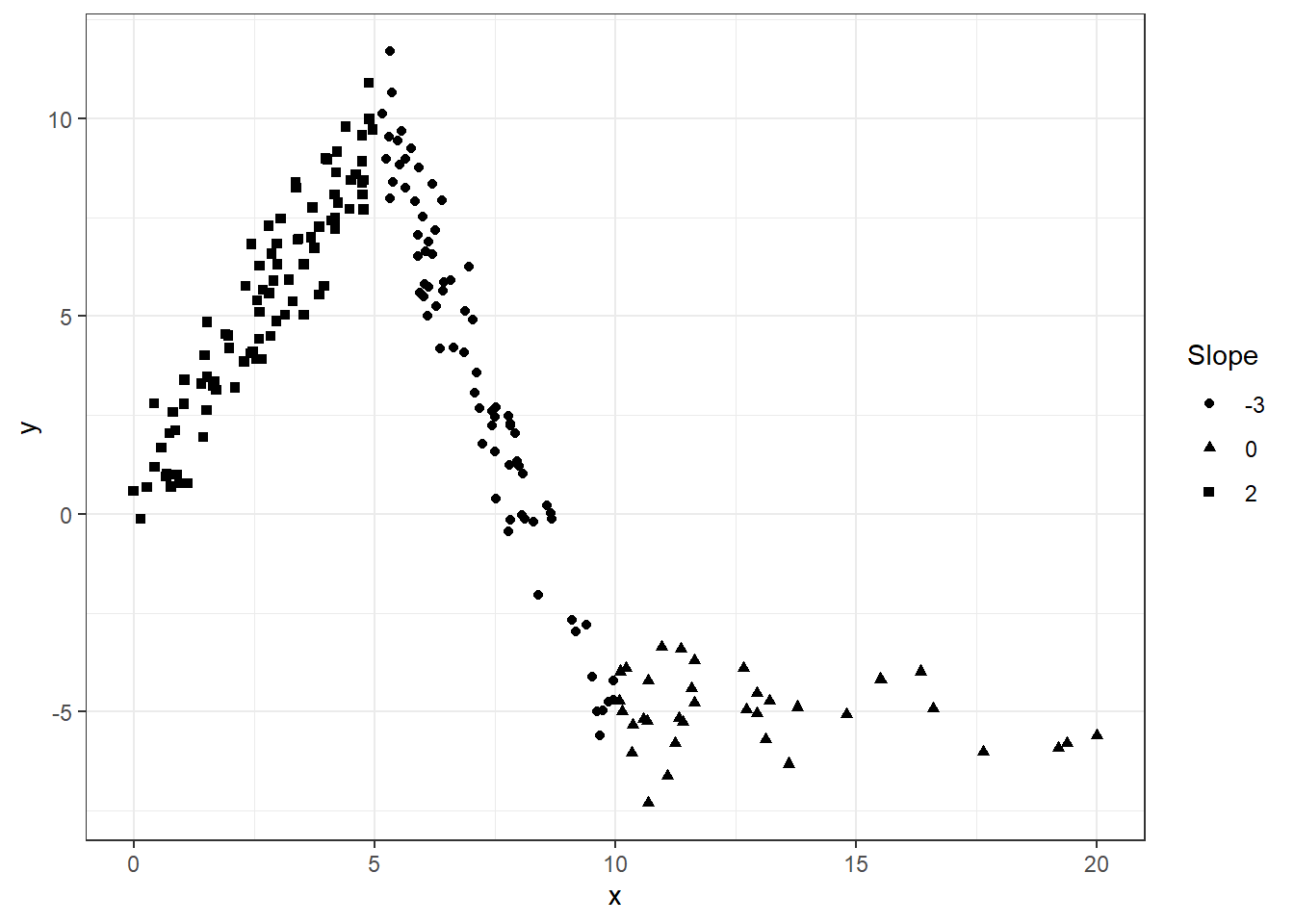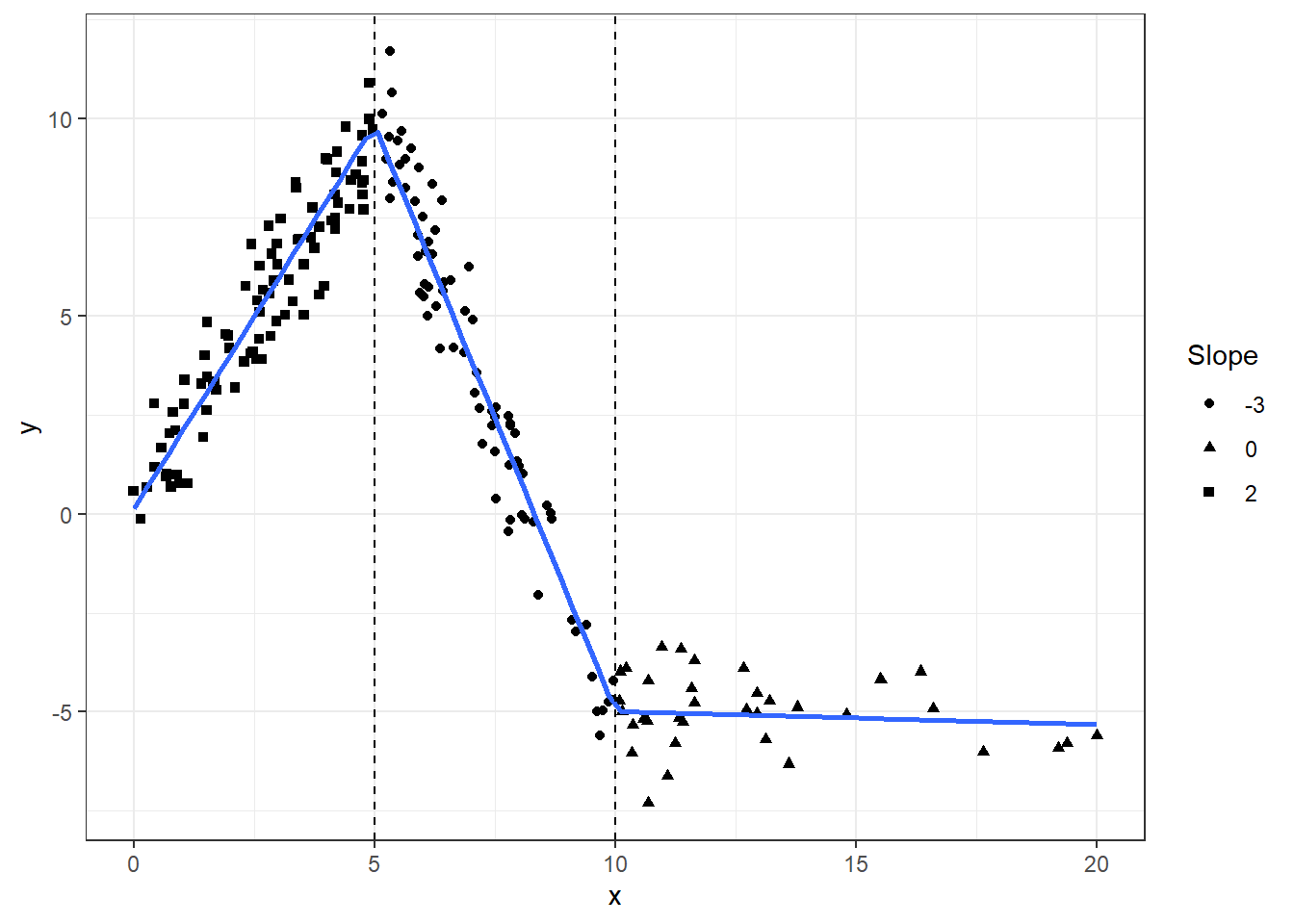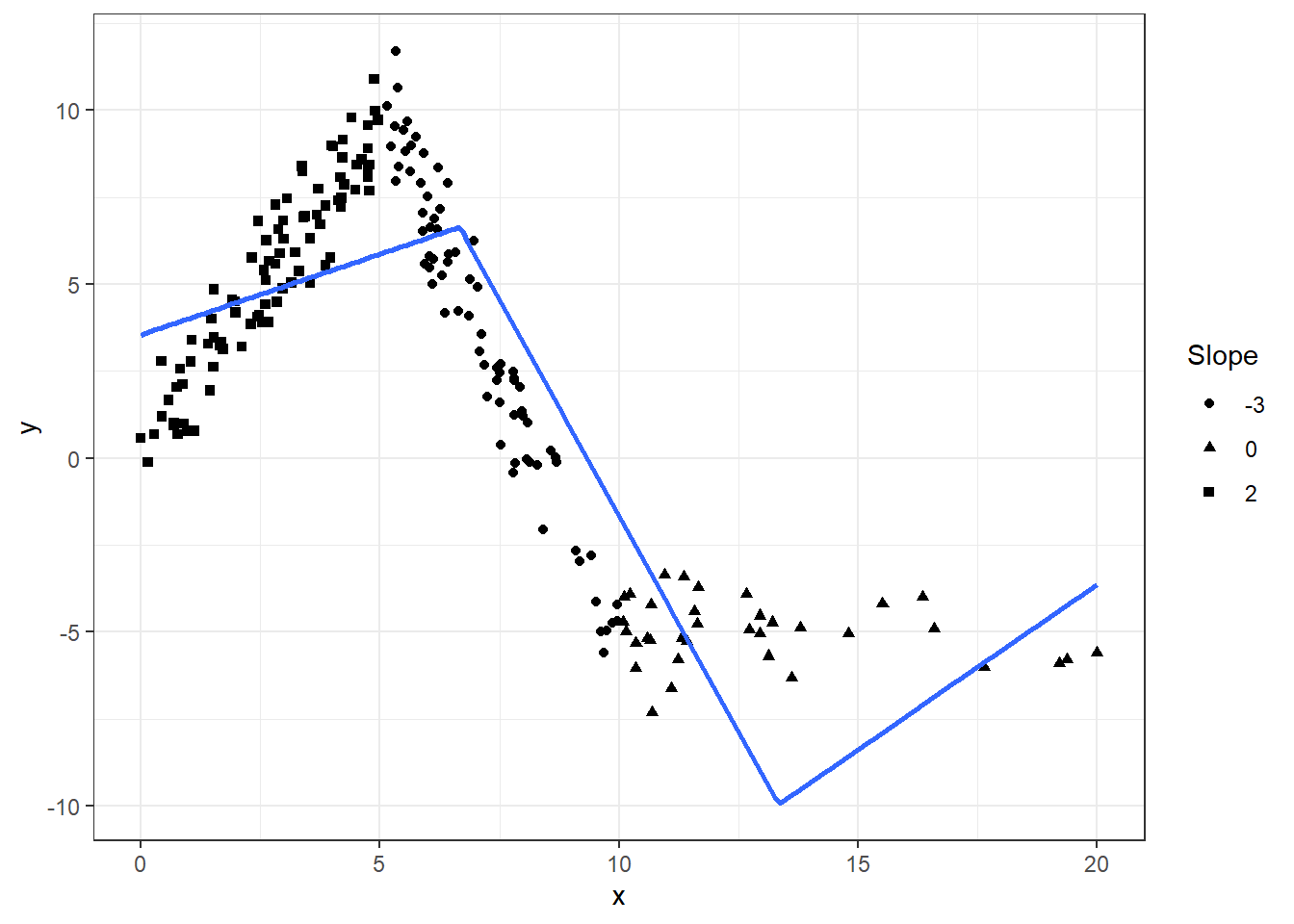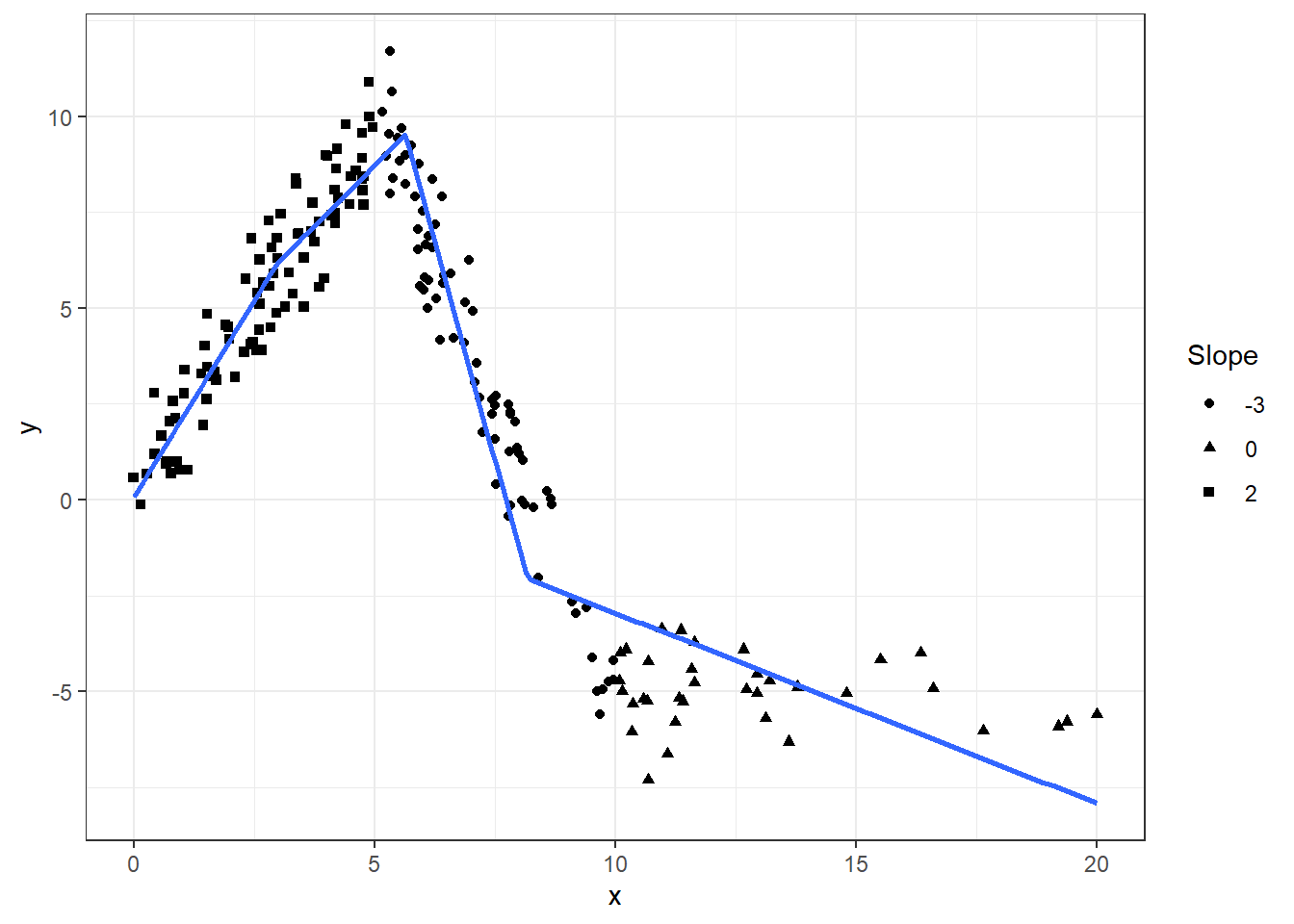I have just published a small package `lspline` on CRAN that implements linear splines using convenient parametrisations such that

• coefficients are slopes of consecutive segments
• coefficients capture slope change at consecutive knots

Knot locations can be specified

• manually (with `lspline()`)
• at breaks dividing the range of `x` into `q` equal-frequency intervals (with `qlspline()`)
• at breaks dividing the range of `x` into `n` equal-width intervals (with `elspline()`)

The implementation follows Greene (2003, chapter 7.5.2).

The package sources are on GitHub here.

# Examples

We will use the following artificial data with knots at `x=5` and `x=10`:

``````set.seed(666)
n <- 200
d <- data.frame(
x = scales::rescale(rchisq(n, 6), c(0, 20))
)
d\$interval <- findInterval(d\$x, c(5, 10), rightmost.closed = TRUE) + 1
d\$slope <- c(2, -3, 0)[d\$interval]
d\$intercept <- c(0, 25, -5)[d\$interval]
d\$y <- with(d, intercept + slope * x + rnorm(n, 0, 1))``````

Plotting `y` against `x`:

``````library(ggplot2)
fig <- ggplot(d, aes(x=x, y=y)) +
geom_point(aes(shape=as.character(slope))) +
scale_shape_discrete(name="Slope") +
theme_bw()
fig``````The slopes of the consecutive segments are 2, -3, and 0.

## Setting knot locations manually

We can parametrize the spline with slopes of individual segments (default `marginal=FALSE`):

``````library(lspline)
m1 <- lm(y ~ lspline(x, c(5, 10)), data=d)
knitr::kable(broom::tidy(m1))``````
termestimatestd.errorstatisticp.value
(Intercept)0.13432040.21481160.62529410.5325054
lspline(x, c(5, 10))11.94354580.059769832.51717470.0000000
lspline(x, c(5, 10))2-2.96667500.0503967-58.86648320.0000000
lspline(x, c(5, 10))3-0.03352890.0518601-0.64652550.5186955

Or parametrize with coeficients measuring change in slope (with `marginal=TRUE`):

``````m2 <- lm(y ~ lspline(x, c(5,10), marginal=TRUE), data=d)
knitr::kable(broom::tidy(m2))``````
termestimatestd.errorstatisticp.value
(Intercept)0.13432040.21481160.62529410.5325054
lspline(x, c(5, 10), marginal = TRUE)11.94354580.059769832.51717470.0000000
lspline(x, c(5, 10), marginal = TRUE)2-4.91022080.0975908-50.31435970.0000000
lspline(x, c(5, 10), marginal = TRUE)32.93314620.088544533.12624790.0000000

The coefficients are

• `lspline(x, c(5, 10), marginal = TRUE)1` – the slope of the first segment
• `lspline(x, c(5, 10), marginal = TRUE)2` – the change in slope at knot x = 5; it is changing from 2 to -3, so by -5
• `lspline(x, c(5, 10), marginal = TRUE)3` – tha change in slope at knot x = 10; it is changing from -3 to 0, so by 3

The two parametrisations (obviously) give identical predicted values:

``all.equal( fitted(m1), fitted(m2) )``
``##  TRUE``

graphically

``````fig +
geom_smooth(method="lm", formula=formula(m1), se=FALSE) +
geom_vline(xintercept = c(5, 10), linetype=2)``````## Knots at `n` equal-length intervals

Function `elspline()` sets the knots at points dividing the range of `x` into `n` equal length intervals.

``````m3 <- lm(y ~ elspline(x, 3), data=d)
knitr::kable(broom::tidy(m3))``````
termestimatestd.errorstatisticp.value
(Intercept)3.54848170.46038277.7076780.00e+00
elspline(x, 3)10.46525070.10102004.6055297.40e-06
elspline(x, 3)2-2.49083850.1167867-21.3281050.00e+00
elspline(x, 3)30.94756300.23286914.0690806.84e-05

Graphically

``````fig +
geom_smooth(aes(group=1), method="lm", formula=formula(m3), se=FALSE, n=200)``````## Knots at `q`uantiles of `x`

Function `qlspline()` sets the knots at points dividing the range of `x` into `q` equal-frequency intervals.

``````m4 <- lm(y ~ qlspline(x, 4), data=d)
knitr::kable(broom::tidy(m4))``````
termestimatestd.errorstatisticp.value
(Intercept)0.07822850.39480610.1981440.8431388
qlspline(x, 4)12.03988040.180272411.3155480.0000000
qlspline(x, 4)21.26751860.14712708.6151320.0000000
qlspline(x, 4)3-4.58464780.1476810-31.0442730.0000000
qlspline(x, 4)4-0.49658580.0572115-8.6798180.0000000

Graphically

``````fig +
geom_smooth(method="lm", formula=formula(m4), se=FALSE, n=200)``````# Installation

Stable version from CRAN or development version from GitHub with

``remotes::install_github("mbojan/lspline", build_vignettes=TRUE)``

# Acknowledgements

Inspired by Stata command `mkspline` and function `ares::lspline()` from Junger & Ponce de Leon (2011). As such, the implementation follows Greene (2003), chapter 7.5.2.

• Greene, William H. (2003) Econometric analysis. Pearson Education
• Junger & Ponce de Leon (2011) `ares`: Environment air pollution epidemiology: a library for timeseries analysis. R package version 0.7.2 retrieved from CRAN archives.